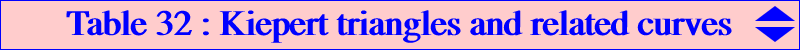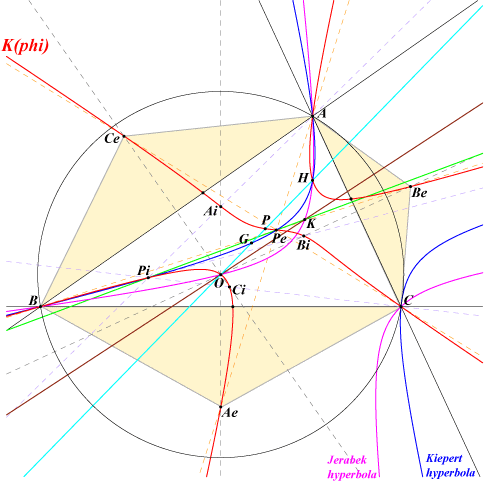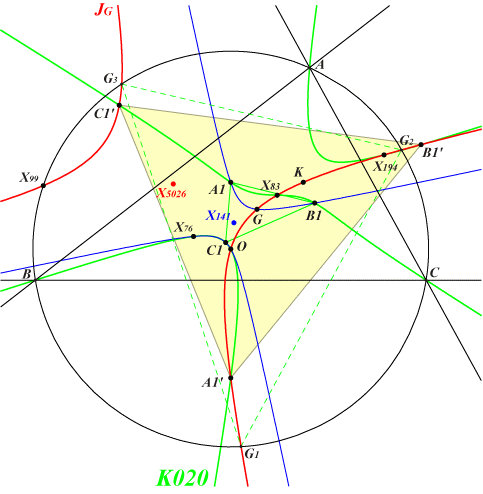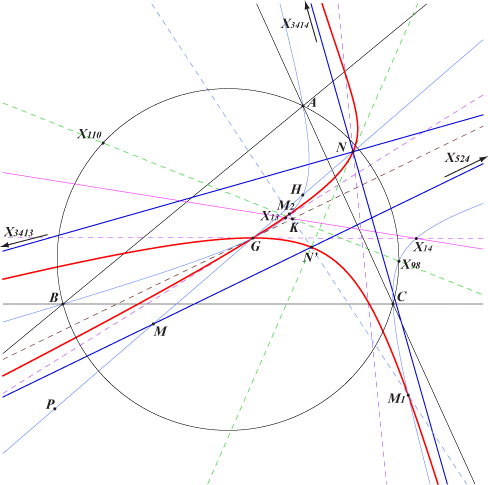The Kiepert triangles are formed by the apices of the isosceles triangles erected on the sides of the reference triangle ABC with the same base angle phi in [–π/2;π/2]. With phi > 0, the triangle is erected outwardly and is denoted AeBeCe and when phi < 0, the triangle is erected inwardly and is denoted AiBiCi. When phi = 0, this is the medial triangle and when phi = +/– π/2, it degenerates into the line at infinity. A Kiepert triangle has always its centroid at G and is always perspective with : – ABC, the perspector lies on the Kiepert hyperbola, – the medial triangle (cevian triangle of G, pedal triangle of O) with perspector O, – the tangential triangle (anticevian triangle of K, antipedal triangle of I) with perspector O again, – the circumcevian and circumanticevian triangles of I (or any excenter) with perspector O again. We generalize these properties and seek, for a given (finite) value of phi > 0, the locus of M whose cevian, pedal, anticevian, antipedal, circumcevian, circumanticevian triangle is perspective with the corresponding Kiepert triangles AeBeCe and AiBiCi.We first recall that there is always an isogonal pK passing through the six apices Ae, Be, Ce and Ai, Bi, Ci of the six triangles obtained with base angle +/– phi. The corresponding perspectors are Pe, Pi on the cubic, on the Kiepert hyperbola and collinear with K. This cubic – here denoted by K(phi) – is a member of the Euler pencil of cubics. Its pivot P lies on the Euler line and is defined by OP = k OH (vectors) where k = (cot^2(phi) + 1) / (3cot^2(phi) – 1). K(phi) contains a large number of points as detailed in Table 27. Obviously, K(phi) = K(–phi).A remarkable special caseWhen phi is the Brocard angle Ω, AiBiCi is the first Brocard triangle A1B1C1 and K(phi) is K020. AeBeCe is called the reflected first Brocard triangle and its vertices are denoted A1', B1', C1'. Its centroid is G, like any other value of phi. The Kiepert hyperbola of this triangle is the Jerabek hyperbola JG of the Grebe triangle. It is perspective to : • the cevian triangle of any P on K421, • the anticevian triangle of any P on K423, • the antipedal triangle of any P on K422. In particular, it is homothetic at X(3972) to the antipedal triangle of X(262). It is orthologic to the antipedal triangle of any point on the Kiepert hyperbola, in particular X(262). One of the centers of orthology lies on JG and the other on another rectangular hyperbola also passing through X(99), X(3413), X(3414). See also the related K797.Kiepert triangles and Cevian triangles Let M be a point and MaMbMc its cevian triangle. The triangles AeBeCe (resp. AiBiCi) and MaMbMc are perspective (at N) if and only if M lies on Cev(phi) (resp. Cev(–phi)), a pK with pole Ωe (resp. Ωi), pivot Qe (resp. Qi). The locus of the perspector N is K(phi) in both cases. With ∆ = area(ABC), the equation of Cev(phi) is : –4∆ F0(x,y,z) + cot(phi) F1(x,y,z) + 4∆ cot^2(phi) F2(x,y,z) + 16 ∆^2 cot^3(phi) F3(x,y,z) =0, where : • F0(x,y,z) = ∑ 2SA x(y^2 - z^2) : F0 = 0 is the Lucas cubic, • F1(x,y,z) = ∑ [(b^2-c^2)^2+a^2(2b^2 +2c^2-3a^2)](y-z)yz : F1 = 0 is the pivotal cubic pK(X20, X2), • F2(x,y,z) = ∑ x [(a^2-b^2+3c^2)y^2 - (a^2+3b^2-c^2)z^2] : F2 = 0 is the Thomson centroidal cubic K295, • F3(x,y,z) = (x-y)(y-z)(z-x) : F3 = 0 is the union of the medians of ABC. We note that the sum of Cev(phi) and Cev(–phi) is pK(P, G), the pivotal cubic with pole the pivot P of K(phi) and pivot G whose equation is given by : – F0(x,y,z) + cot^2(phi) F2(x,y,z) = 0. *** These two cubics Cev(phi) and Cev(–phi) coincide when : – phi = +/– π/2 and the locus is the Lucas cubic (since this cevian triangle must be a pedal triangle) and the perspector lies on the Darboux cubic. – phi = 0, the locus is the union of the medians. Hence, in all other cases, each Euler cubic K(phi) can be associated with the two cubics Cev(phi) and Cev(–phi) which we call the Kiepert Cevian Mates of K(phi). Construction of points on Cev(phi) and Cev(–phi) For any point N on K(phi), the lines AeN, BeN, CeN meet BC, CA, AB at three points forming a triangle perspective with ABC and the perspector is M = Fe(N) on Cev(phi). A similar construction is obtained for Cev(–phi) with the points Ai, Bi, Ci. Note that P, M, N lie on same line which also contains N* (isogonal conjugate of N, on K(phi)) and Fe(N*) on Cev(phi). Properties of Cev(phi) = pK(Ωe, Qe) [and likewise for Cev(–phi)] – the pivot is Qe = Fe(P) where P is the pivot of K(phi). – the isopivot is Pe. – the pole Ωe lies on the Kiepert hyperbola. It is the barycentric product of Pe and Qe. – the cubic passes through G (since the medial triangle is perspective to any Kiepert triangle). – the cubic contains its pole Ωe, since it is the isoconjugate of G. – the cubic contains P. It follows many collinearities between all these points : – G, Ωe, Qe, Ωi* and G, Ωi, Qi, Ωe* (M* denotes the isogonal conjugate of M). – K, Ωe, Ωi and K, Pe, Pi, P/O (P/O is the cevian quotient of P and O. It lies on the line OP*, on K(phi) and on the Stammler hyperbola). – P, P*, Qe, Qi. – P, Ωe, Pi and P, Ωi, Pe. More over, the lines PeQe, PiQi intersect at X on the Kiepert hyperbola and the lines PeΩe, PiΩi intersect at Y on the Euler line. Y is the harmonic conjugate of P with respect to G and H. Kiepert Cevian Mates of usual cubics For several simple values of phi, we obtain most of the well known cubics of the Euler pencil. The table gives these cubics with their mates.phi K(phi) Cev(phi) Cev(–phi) Remarks π/6 K005 K420b K420a π/4 K006 K070b K070a These are the Shoemaker's Cubics π/3 K001 K419b K419a π/2 K004 K007 Ω K020 K421 K322 K421 is the only Cev(phi) through K, Ω is the Brocard angleThe equation given above clearly shows that, in general, there are three cubics Cev(phi) passing through a given point Q distinct of G and P but, when Q is O or K, one can find only one such cubic. In particular, – the cubics K419a, K419b, K322 are those passing through the infinite points of the Steiner ellipse, – the cubics K007, K070a, K070b are those passing through H, – the cubics K419a, K419b, K420b contain X(13) and the cubics K419a, K419b, K420a contain X(14), – the cubics K420b and Cev(π/12), Cev(–5π/12) contain X(17) and the cubics K420a and Cev(–π/12), Cev(5π/12) contain X(18),Kiepert triangles and Anticevian triangles Let M be a point and MaMbMc its anticevian triangle. The triangles AeBeCe (resp. AiBiCi) and MaMbMc are perspective (at N) if and only if M lies on Acv(phi) (resp. Acv(–phi)), an isogonal pK with pivot Qe (resp. Qi) on the line GK. The locus of the perspector N is K(phi) again in both cases. With ∆ = area(ABC), the equation of Acv(phi) is : ∆ (1 + cot^2(phi)) ∑a^2(y–z)yz + cot(phi) ∑a^2(c^2y–b^2z)yz = 0, or equivalently : 2∆ ∑a^2(y–z)yz + sin(2 phi) ∑a^2(c^2y–b^2z)yz = 0. This clearly shows that : – all these cubics belong to the pencil generated by the Thomson cubic K002 and the Grebe cubic K102. See Table 13. Hence, each cubic contains A, B, C, the in/excenters, G and K. – two complementary values of phi give the same cubic i.e. Acv(phi) = Acv(π/2 – phi) although the locus of the perspector is not the same. Thus, it is enough to take phi in [0 ; π/4] to obtain all the cubics of the pencil. *** These two cubics Acv(phi) and Acv(–phi) coincide when phi = 0 : the locus is the Thomson cubic and the perspector lies on the Darboux cubic. Hence, in all other cases, each Euler cubic K(phi) can be associated with the two cubics Acv(phi) and Acv(–phi) which we call the Kiepert AntiCevian Mates of K(phi). Properties of Acv(phi) = pK(X6, Qe) and Acv(–phi) = pK(X6, Qi) – There is one and only one cubic passing through another point Q not in the basis of the pencil. – Acv(phi) contains Pe (perspector of ABC and AeBeCe) and its isogonal conjugate Pe*. Similarly, Acv(–phi) contains Pi and Pi*. – The pivot Qe of Acv(phi) is the intersection of GK and PPe. Qe and Qi are harmonic conjugates with respect to G and K. – AeBeCe and the excentral triangle are always perspective and the perspector is X(4)X(9) /\ X(1)Pe*. – AeBeCe and the antimedial triangle are always perspective and the perspector lies on the line through G, Pe*, Pi and on the Steiner rectangular hyperbola. – AeBeCe and the tangential triangle are always perspective and the perspector is O. Kiepert AntiCevian Mates of usual cubics For several simple values of phi, we obtain most of the well known cubics of the Euler pencil. The table gives these cubics with their mates.phi K(phi) K(π/2–phi) Acv(phi) Acv(–phi) Remarks 0 K002 K004 K002 π/12 pK(X6, X590) pK(X6, X615) π/6 K005 K001 K129b K129a π/4 K006 K424b K424a Ω K020 K422 K423 K128 Ω is the Brocard angleLoci related to centers of Kiepert triangles This section written with the cooperation of Chris van Tienhoven Let A'B'C' be a Kiepert triangle and let P' be a center of A'B'C'. Let P be this same center in ABC. We seek the locus of P' when A'B'C' varies i.e. when the base angle phi varies in [–π/2;π/2]. This locus will be called the P-Kiepert locus. The general case seems out of reach and we shall concentrate on several particular cases, those related to the most common (and the most simple) centers of ABC. P-Kiepert loci related to P on the Euler line Let us consider a center P on the Euler defined by GP = k GO (vectors) where k is any real number. Recall that when P = G (i.e. k = 0) we always have P' = G. For a given value of k, the P-Kiepert locus – here denoted T(k) – of P' is a nodal cubic with node G. These cubics form a pencil that is generated by the two following limit cases : • when k->0, T(k) is the union of the line GK and the axes of the Steiner ellipses, • when k->∞, T(k) is the union of the line at infinity and the two lines passing through G and the Fermat points X(13), X(14). It follows that • T(k) has three real asymptotes with infinite points those of T(k->0) namely X(524), X(3413), X(3414). These two latter points are the infinite points of the axes of the Steiner ellipses and also the infinite points of the Kiepert hyperbola. More precisely, the asymptote passing through X(524) – therefore parallel to the line GK – meets the Euler line at the midpoint M of GP and the two other asymptotes meet at N, the complement of P. N lies on the cubic T(k) and the tangent at N is parallel to the line HK. The tangential N' of N is the intersection of this latter tangent with the first asymptote. • The nodal tangents at G are the lines passing through the Fermat points. • T(k) meets the Kiepert hyperbola at G (twice), X(3413), X(3414) and two other points M1, M2 that lie on the line KN'.The following table gives a selection of these cubics. The four points X(2), X(524), X(3413), X(3414) are not repeated. The red point is N, the blue point is N', the green points are M1, M2.k P centers on T(k) T(k) 1 X(3) X(5), X(182), X(627), X(628), X(641), X(642), etc. K801 -2 X(4) X(3), X(485), X(486), X(1352), X(1689), X(1690) anticomplement of K801 -1/2 X(5) X(17), X(18), X(140) complement of K801 4 X(20) X(4), X(487), X(488)